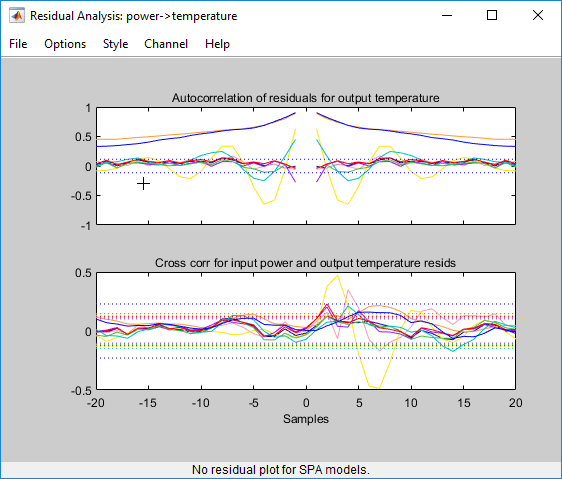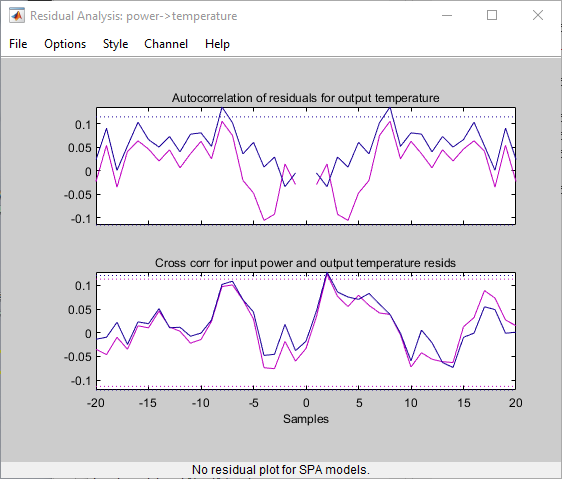## Examine Model Residuals

This example shows how you can use residual analysis to evaluate model quality.

### Creating Residual Plots

1. To load the sample System Identification app session that contains estimated models, type the following command in the MATLAB® Command Window:

`systemIdentification('dryer2_linear_models')`
2. To generate a residual analysis plot, select the Model resids check box in the System Identification app.

This opens an empty plot.

3. In the System Identification app window, click each parametric model icon to display it on the Residual Analysis plot. Do not click the nonparametric model `spad`, as residual analysis plots are not available for this model.### Description of the Residual Plot Axes

The top axes show the autocorrelation of residuals for the output (whiteness test). The horizontal scale is the number of lags, which is the time difference (in samples) between the signals at which the correlation is estimated. The horizontal dashed lines on the plot represent the confidence interval of the corresponding estimates. Any fluctuations within the confidence interval are considered to be insignificant. Six of the models —`arxqs`, `n4s3`, `arx223`, `tf1`,`ss1`, and `amx2222` — produce residuals that enter outside the confidence interval. A good model should have a residual autocorrelation function within the confidence interval, indicating that the residuals are uncorrelated.

The bottom axes show the cross-correlation of the residuals with the input. A good model should have residuals uncorrelated with past inputs (independence test). Evidence of correlation indicates that the model does not describe how the output is formed from the corresponding input. For example, when there is a peak outside the confidence interval for lag k, this means that the contribution to the output y(t) that originates from the input u(t-k) is not properly described by the model. The models `arxqs` and `amx2222` extend beyond the confidence interval and do not perform as well as the other models.

### Validating Models Using Analyzing Residuals

To remove models with poor performance from the Residual Analysis plot, click the model icons `arxqs`, `n4s3`, `arx223`, `tf1`, `ss1`, and `amx2222` in the System Identification app.

The Residual Analysis plot now includes only the two models that pass the residual tests: `arx692` and `amx3322`.The plots for these models fall primarily within the confidence intervals, although the `amx3322` plot shows some excursions slightly outside of the boundaries. If these excursions are acceptable, it is reasonable to pick the `amx3322` model because it is a simpler low-order model. If the excursions are not acceptable, you can use the `arx392` model instead.

## Related Examples